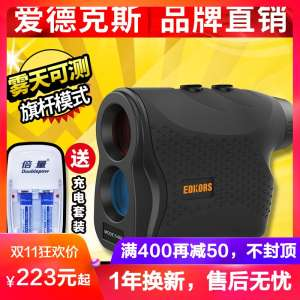# Handheld laser range finder telescope 600 meters 1000 meters 1500 meters outdoor measurement angle measuring distance telescopeID: 131740000030 Price 39.72\$ Sold/Remain 323/5756 Factory Factory direct outlet link

EDKORS / Edwards

LD

#### Item:

Color Classification:

#### 600 meters range (basic models) 600 meters range measurement height measurement + continuous measurement + golf mode + two-point height of 1000 meters ranging + continuous measurement + golf mode 1000 meters range measurement height measurement + continuous measurement + golf Mode + two-point height measurement 1500 meters ranging + continuous measurement + golf mode 1500 meters range measurement height measurement + continuous measurement + golf mode + two-point measurement 600 meters ranging + continuous measurement + golf mode

Decoration and construction content:

5-1500M

20170413

#### laser

Distance: